Courses

# Test: Algebraic Expressions - 3

## 20 Questions MCQ Test Mathematics (Maths) Class 7 | Test: Algebraic Expressions - 3

Description
This mock test of Test: Algebraic Expressions - 3 for Class 7 helps you for every Class 7 entrance exam. This contains 20 Multiple Choice Questions for Class 7 Test: Algebraic Expressions - 3 (mcq) to study with solutions a complete question bank. The solved questions answers in this Test: Algebraic Expressions - 3 quiz give you a good mix of easy questions and tough questions. Class 7 students definitely take this Test: Algebraic Expressions - 3 exercise for a better result in the exam. You can find other Test: Algebraic Expressions - 3 extra questions, long questions & short questions for Class 7 on EduRev as well by searching above.
QUESTION: 1

### If x+y = 5, y+z = 7and z+x = 12, what is the value of x+y+z?

Solution: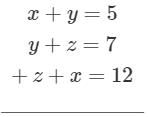⇒ x+y+z = 12

QUESTION: 2

### How many auxiliary formulae can be formed from the expression in the box?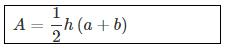Solution:

3 formulae with the three unknowns can be formed from the given expression.

QUESTION: 3

### What is the difference between 3a + 2b and −2a − 5b?

Solution:

(3a + 2b) − (−2a − 5b)
= 3a + 2b + 2a + 5b = 5a + 7b

QUESTION: 4

The length and breadth of a rectangular plot are I and b. Two rectangular paths each of width W run inside the plot one parallel to the length and the other parallel to the breadth. What is the total area of the paths?

Solution: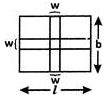Area of the path along length = l×w
Area of the path along breadth = l×w
The common area of the paths = w2
∴ Total area of the path = lw+lw−w2 = (l+b−w)w

QUESTION: 5

In a two digit number, the units digit is x and tens digit is (x+3). What is the sum of the digits in the number?

Solution:

Sum of units digit (x) and tens digit (x + 3) is x + x + 3 = 2x+3.

QUESTION: 6

A and B are polynomials and each is the additive inverse of the other. What does it mean?

Solution:
QUESTION: 7

When a certain number, 'm' is divided by 5 and added to 8, the result is equal to thrice the number subtracted from 4. What is the value of 'm?

Solution:
QUESTION: 8

Find the value of the expression x + 2 for x = -2

Solution:

-2 + 2 = 0

QUESTION: 9

In the figure given what is the perimeter, in cm, of the triangle?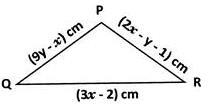Solution:
QUESTION: 10

If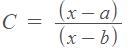, find the value of x.

Solution:⇒ C(x−b) = x−a
⇒ x =QUESTION: 11

Match the following.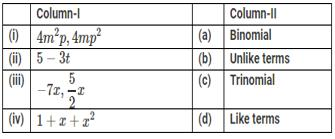Solution:

The correct option is Option B.

(i)-(b) : 4m²p and 4mp² are unlike terms

(ii)-(a) : 5 - 3t is a binomial

(iii)-(d) : -7x, 5/2x are like terms

(iv)-(c) : 1 + x + x² is a trinomial

QUESTION: 12

Which of the following is true?

Solution:
QUESTION: 13

If x = 3, a = (−1) and b= (−2), what is the value of 2−6x + 4a−3b?

Solution:
QUESTION: 14

For what value of 'm' is 9 − 5m = (−1)?

Solution:
QUESTION: 15

Simplify x2y−1.5x2y3+1.4x2y3.

Solution:
QUESTION: 16

What is the value of(a3−2a2+4a−5)−(−a3−8a+2a2+5)?

Solution:

a3−2a+ 4a − 5 −(−a− 8a + 2a2+5)=a− 2a+ 4a − 5 + a+ 8a - 2a2-5
=2a− 4a+ 12a − 10

QUESTION: 17

By how much is x4−4x2y2+yless than x4+8x2y2+y4?

Solution:

x4+8x2y2+y4-(x4-4x2y2+y4)=x4+8x2y2+y4-x4+4x2y2-y4
=12x2y2

QUESTION: 18

What is the sum of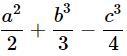;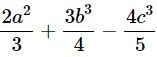and a2+b3+c3?

Solution: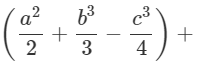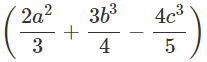+ (a+ b+ c3)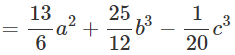QUESTION: 19

Match the following.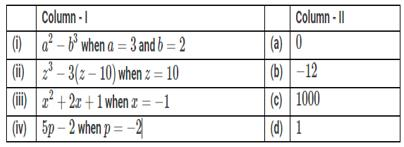Solution:

Substitute the given values in the expressions and evaluate.

a3−b= 33−2= 9−8 =1

z3−3 (z−10) =103−3(10−10)

=10− 0 =1000

x2+2x+1 = (−1)+ 2(1)+1

= 1−2+1 = 0

QUESTION: 20

Simplify the following expression.x(y−z)−y(z−x)−z(x−y)

Solution:

x(y−z) − y(z−x) − z(x−y)
= xy − xz − yz + xy − zx + yz
= 2xy − 2zx = 2x(y−z)